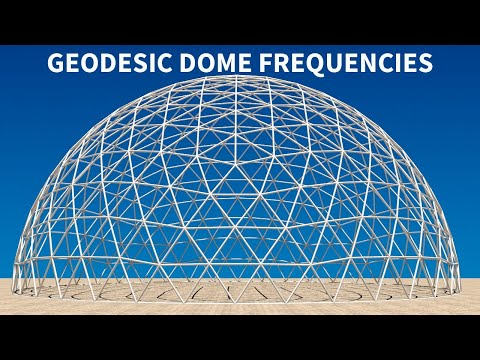Geodesic Domes Frequencies | Pacific Domes

You are here:

# Geodesic Domes Frequencies

Knowledge Base | Geodesic Domes Frequencies

# Geodesic Dome Frequencies

When talking about domes, the definition of frequency refers to the number of pieces that each edge of the base figure is divided into in the process of triangulating its sides. For instance, we might start with a base figure of an icosahedron and divide each edge of each triangular face into 3 equal lengths. Those new points are connected to divide the original triangle into 9 smaller triangles. Since the original edges were divided into 3 parts, we call this a 3-frequency dome. The frequency is commonly abbreviated as “v”, so a 2-frequency dome is called 2v, 3-frequency is 3v, and so forth. The higher the frequency the more curved and sphere like the dome appears.

## 8 Frequency 120ft (37m) Domes